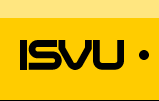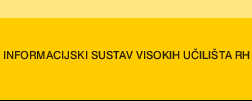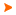Podaci o VU Početna stranicaArhiva nastavnih programaArhiva ak. kalendaraAbbreviation: B23A02 Load: 30(L) + 0(P) + 0(FE) + 0(LE) + 0(S) + 0(PEE) + 30(E) + 0()
 Lecturers in charge: doc. dr. sc. Jelka Beban-Brkić Lecturers: v. pred. mr. sc. Željka Tutek ( Exercises ) Course description: To recognize the mathematical and numerical skills acquired within the theory of curves and surfaces in the field of study. To use the mathematical and numerical skills acquired within the theory of curves and surfaces for solving problems in the field of study.Learning outcomes at the level of the programme to which the course contributesUnderstand mathematical methods and physical laws applied in geodesy and geoinformatics.Apply knowledge of mathematics and physics for the purpose of recognizing, formulating and solving of problems in the field of geodesy and geoinformatics.Use information technology in solving geodetic and geoinformation tasksExercise appropriate judgements on the basis of performed calculation processing and interpretation of data obtained by means of surveying and its results.Take responsibility for continuing academic development in the field of geodesy and geoinformatics, or related disciplines, and for the development of interest in lifelong learning and further professional education.Learning outcomes expected at the level of the courseidentify various forms of curve equations, calculate arc length, curvature and determine the associated vector fields; Identify and differentiate between types of second order surfaces;analyze the second order surfaces with emphasis on the sphere and the ellipsoid of revolution: determine the parameter curves, the tangent plane and the normal vector to the surface;determine the first fundamental form of the surface and use it to calculate arc length, surface area and angle between two curves on a surface;determine the second fundamental form of the surface and use it for classifying points on the surface, calculating the normal, principal, Gaussian and mean curvature of the surface;detect some special curves on surfaces (lines of curvature, asymptotic lines);define the concept of the geodesic curvature along a curve on a surfaces and the term geodesic; calculate the geodesic curvature of parameter curves in order to identify whether it is a matter of geodesic coordinates;pronounce the Theorema Egregium of Gauss;distinguish and name types of mappings of surfaces according to the mapping invariants;use a variety of tools for visualizing and solving problems related to the theory of curves and surfaces.Course content broken down in detail by weekly class schedule (syllabus)Basic concepts of vector algebra and vector analysis. 1hRepresentations of curves. 1hArc length and re-parameterisation of a curve. 2hMoving trihedron. Curvature and torsion. Frenet-Serret formulas. 2hConcept of a surface: definition, parametric representation, coordinate patches, parameter curves. 2hConcept of a surface: the tangent plane and the normal vector to the surface. 2hReview of previous work. 1h1st preliminary exam 1hFirst fundamental form and its applications (arc length, surface area and angle between two curves on a surface). 2hSecond fundamental form and its applications (normal curvature, types of points on the surface). 2hAsymptotic and principal directions and lines. Principal, Gaussian and mean curvature of the surface. 2hEuler's theorem and Dupin's indicatrix. 1hGauss-Weingarten equations and Christoffel symbols. The fundamental theorem of surfaces. 2hReview of previous work. 1h2nd preliminary exam 1hGeodesics (geodesic curvature, geodesic coordinates, arcs of minimum length) 3hMappings of surfaces (Stereographic projection, Mercator projection) 2hReview of previous work. 1hThe final exam. 1hScreening student workClass attendance - Requirement for the signatureindependent assignments - 15%interactive tasks - 5%Tests - 80%Oral exam - optionalWritten exam - 100%
 Lecture languages: - - -
 Compulsory literature: 1. Beban Brkić, J.: Diferencijalna geometrija, Interna skripta, dostupna na e-učenju 2. Žarinac-Frančula, B.: Diferencijalna geometrija, Zbirka zadataka i repetitorij. Školska knjiga, Zagreb 1990.
 Recommended literature: 3. Lipschutz, M. M.: Differential Geometry, Schaum's Outline Series, McGraw-Hill Book Company, N. Y. 1969. 4. Gray, A.: Modern Differential Geometry of Curver and Surfaces With Mathematica, CRS Press, Boston, London, 1998.
 Prerequisit for enrollment: Completed : Vector Analysis Passed : Analytical Geometry and Linear Algebra Passed : Mathematical AnalysisLegend L - Lectures P - Practicum FE - Field exercises LE - Laboratory exercises S - Seminar PEE - Physical education excercises. E - Exercises - * - Not graded| Početna stranica | Visoka učilišta | Korisničke stranice | | Na vrh | Copyright (c) 2006. Ministarstva znanosti, obrazovanja i športa. Sva prava zadržana. Programska podrška (c) 2006. Fakultet elektrotehnike i računarstva. Oblikovanje(c) 2006. Listopad Web Studio. Posljednja izmjena 2016-07-27